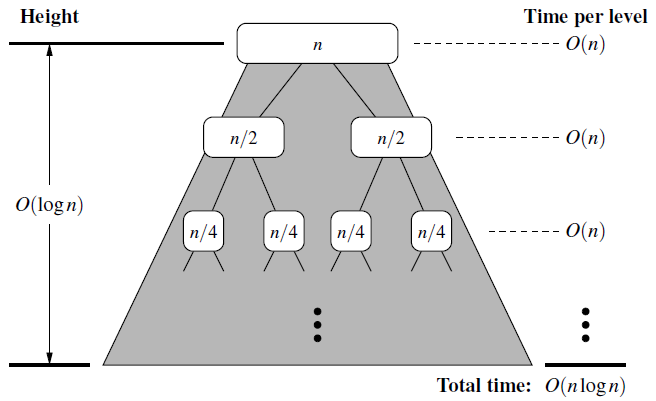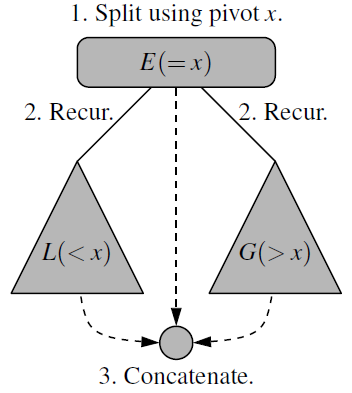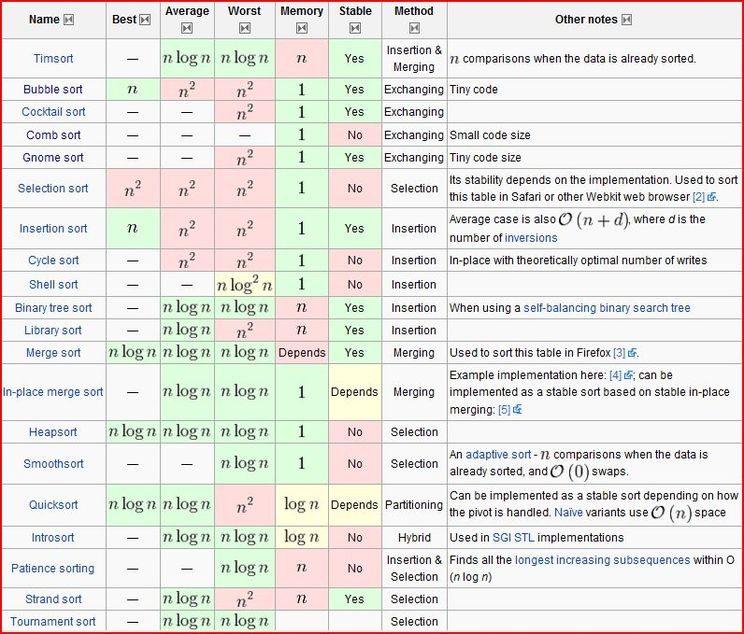Learn about merge-sort, quick-sort, other sorting algorithms and their running time.

# Merge-Sort

• Merge-Sort uses divide-and-conquer algorithmic design pattern to sort a sequence S with n elements.

## Divide-and-Conquer

1. Divide: If the input size is smaller than a certain threshold, solve the problem directly using a straightforward method and return the solution. Otherwise, divide the input data into two or more disjoint subset.
2. Conquer: Recursively solve the subproblems associated with the subsets.
3. Combine: Take the solutions to the subproblems and merge them into a solution to the original problem.

## Implementation

# In Python
# Merge two sorted Python lists S1 and S2 into properly sized list S
def merge(S1, S2, S):
i = j = 0
while i + j < len(S):
if j == len(S2) or (i<len(S1) and S1[i] < S2[j]):
S[i+j] = S[i] # copy ith element of S1 as next item of S
i += 1
else:
S[i+j] = S2[j] # copy jth element of S2 as next item of S
j += 1

# Sort the elements of Python list S using the merge-sort algorithm
def merge_sort(S):
n = len(S)
if n<2: return # list is already sorted

# Divide
mid = n // 2
S1 = S[0:mid] # copy of first half
S2 = S[mid:n] # copy of second half

# Conquer (with recursion)
merge_sort(S1) # sort copy of first half
merge_sort(S2) # sort copy of second half

# Merge results
merge(S1, S2, S) # merge sorted halves back into S


## Running Time

• Algorithm merge-sort sorts a sequence S if size n in $O(n\log n)$ time, assuming two elements of S can be compared in $O(1)$ time.# Quick-Sort

## Description of Quick-sort

• Quick-Sort algorithm uses divide-and-conquer technique to sort a sequence S using a simple recursive approach.
1. Divide: If $S$ has at least two elements, select a specific element $x$ from S, which is called the pivot. Remove all the elements from $S$ and put them into three sequences:
• $L$, storing the elements in $S$ less than $x$
• $E$, storing the elements in $S$ equal to $x$
• $G$, storing the elements in $S$ greater than $x$
2. Conquer: Recursively sort sequences $L$ and $G$.
3. Combine: put back the elements into $S$ in order by first inserting the elements of $L$, then those of $E$, and finally those of $G$.## Implementation

# In Python
# Sort the elements of queue S using the quick-sort algorithm
def quick_sort(S):
n = len(S)
if n<2: return # list is already sorted

# Divide
p = S.first() # using first as arbitrary pivot
while not S.is_empty(): # divide S into L, E, and G
if S.first() < p
L.enqueue(S.dequeue())
elif p < S.first():
G.enqueue(S.dequeue())
else:
E.enqueue(S.dequeue())

# Conquere (with recursion)
quick_sort(L) # sort elements less than p
quick_sort(G) # sort elements greater than p

# Concatenate results
while not L.is_empty():
S.enqueue(L,dequeue())
while not E.is_empty():
S.enqueue(E.dequeue())
while not G.is_empty():
S.enqueue(G.dequeue())


## Running Time

• Quick-Sort runs in $O(n\log n)$ time, and $O(n^2)$ in the worst-case.
• Randomized Quick-Sort gives $O(n\log n)$ expected running time.
• Memory: $O(\log n)$

# Bubble Sort

• In bubble sort, we start at the beginning of the array and swap the first two elements if the first is greater than the second
• Then, we go to the next pair, and so on, continuously making sweeps of the array until it is sorted.
• Runtime: $O(n^2)$ average and worst case, Memory: $O(1)$.

# Selection Sort

• Selection sort is simple but inefficient.
• Find the smallest element using a linear scan and move it to the front (swapping it with the front element).
• Continue doing this until all the elements are in place

# Insertion Sort

• Insertion sort is relatively efficient for small lists and mostly sorted list.
• It works by taking elements from the list one by one and inserting them in their correct position into a new sorted list.
• Runtime: $O(n^2)$, Memory: $O(1)$

# Heap Sort

• Heap sort works by determining the largest (or smallest) element of the list, placing that at the end (or beginning) of the list.
• Then, continuing with the rest of the list by using a data structure heap (a special type of binary tree).
• Runtime: $O(n\log(n))$, Memory: $O(1)$

• Radix sort is a sorting algorithm for integers that takes advantage of the fact that integers have a finite number of bits.
• We iterate through each digit of the number, grouping numbers by each digit.
• Then, we sort each of these groupings by the next digit.
• Runtime: $O(kn)$ ($n$ is the number of elements and $k$ is the number of passes of the sorting algorithm)

# Comparison and Non-comparison Sort

## Comparison Sort

• Comparison sort is a type of sorting algorithm that only reads the list elements through a single comparison operation and determines which of two elements should occur first in the final sorted list.
• Comparison: $% $
• Examples : Bubble sort, Insertion sort, Selection sort, Quick sort, Heap sort, Merge sort, Odd-even sort, Cocktail sort, Cycle sort, Merge insertion sort, Smoothsort, Timsort
• Limitations of Comparison Sorting
• To sort $n$ elements, comparison sorts must make $\Omega(n\log n)$ comparisons in the worst case.
• That is a comparison sort must have lower bound of $\Omega(n\log n)$ comparison operations, which is known as linear or linearithmic time.

## Non-comparison Sort

• Non-comparison sort perform sorting without comparing the elements rather by making certain assumptions about the data.
• Examples:
1. Counting sort (indexes using key values)
2. Radix sort( examines individual bits of keys)
3. Bucket sort( examines bits of keys)
• These are linear sorting algorithms.
• They make certain assumptions about the data.

# Sorting Algorithm Comparison• Book: Cracking the coding interview [Link]
• Book: Data structures and algorithms in python [Link]